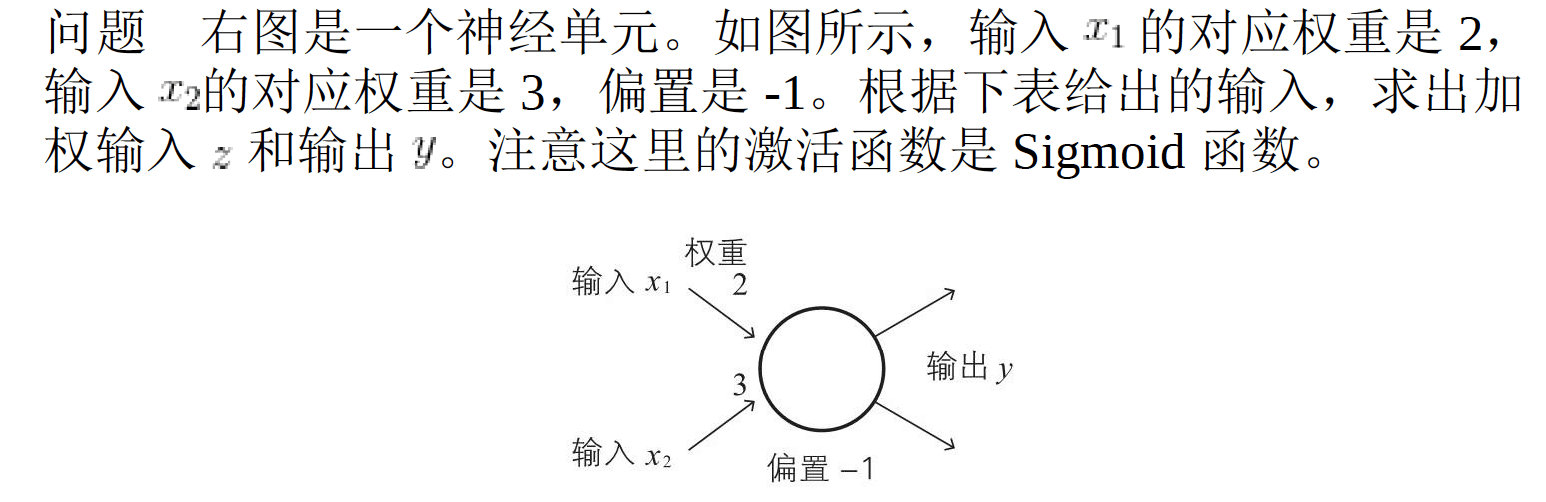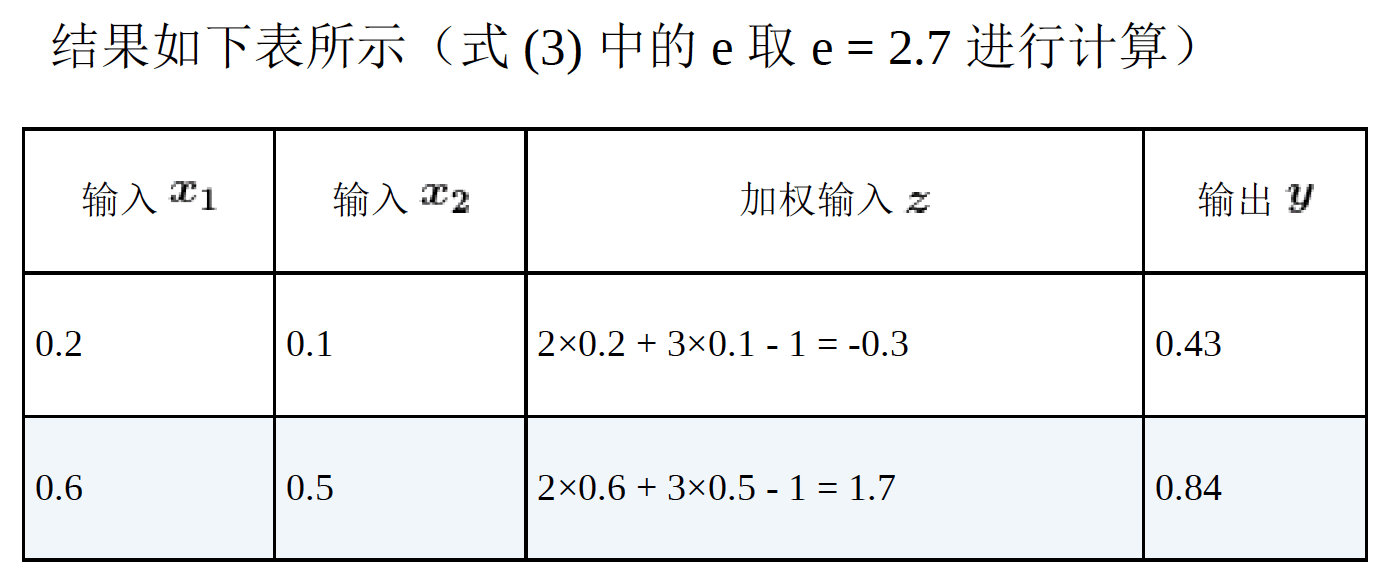# 深度学习数学基础(一)之神经元构造

Posted by Naah on Thursday, Feb 06,2020 10:46:49

# 1 生物学上的神经元

## 1.1 神经元的概念

1. 神经元形成网络
2. 从其他多个神经元传递过来的信号，如果他们的和不超过某个固定大小的值（阈值），则神经元不做出任何反应
3. 如果他们的和超过某个固定大小的值（阈值），则神经元做出反应（称为点火），向另外的神经元传递固定强度的信号
4. 在2和3中，从多个神经元传递过来的信号中，每个信号对应的权重是不同的。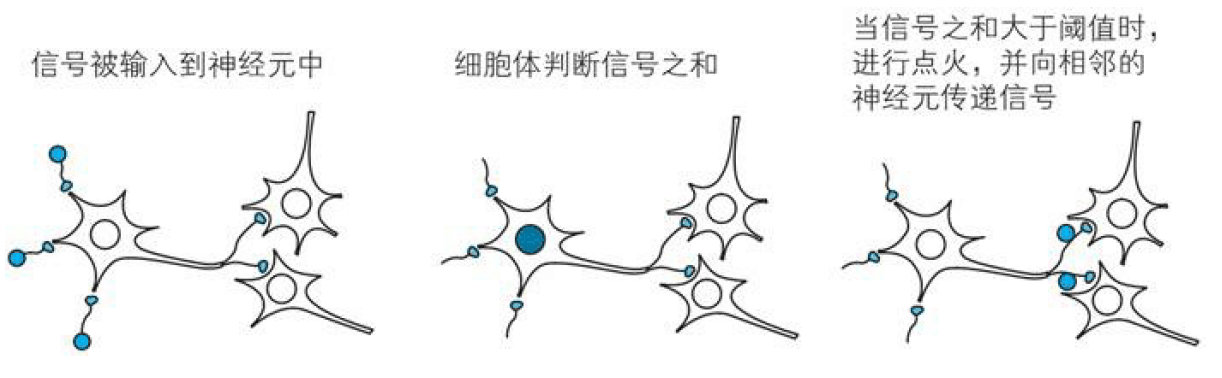## 1.2 神经元的工作

（想想网络上说的玻璃心是不是就是这个意思呢）

# 2 神经元的简单数学表示

1. 来自其他多个神经元的信号之和成为神经元的输入
2. 如果输入超过神经元固有的阈值，则点火
3. 神经元的点火输出可以用数字信号0和1来表示，及时有多个输出端，值也是同一个。

## 2.1 输入## 2.2 输出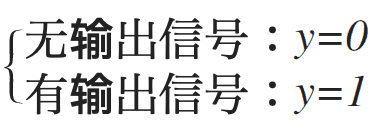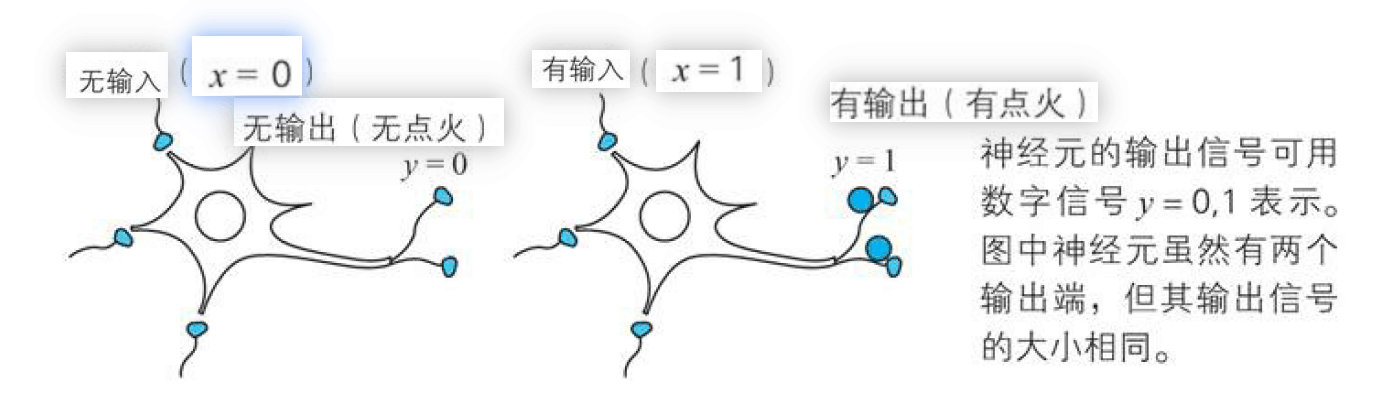## 2.3 权重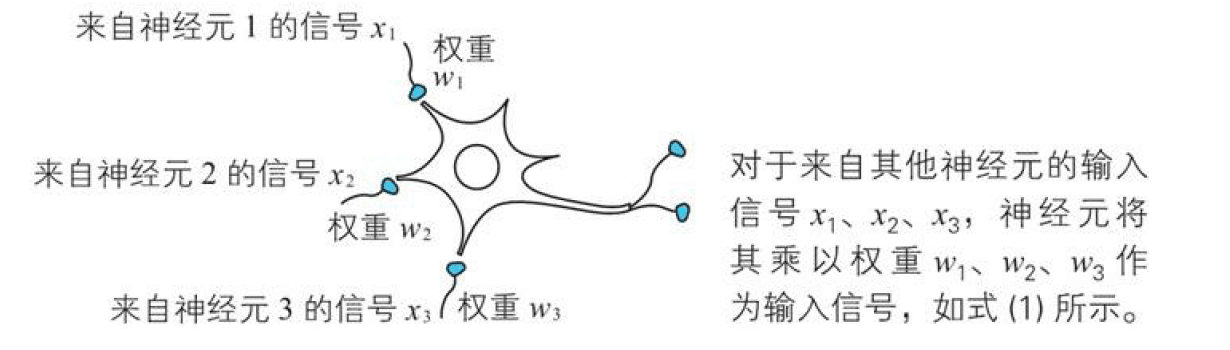## 2.4 点火条件

$$无输出信号（y=0）：{w}_{1}{x}_{1}+{w}_{2}{x}_{2}+{w}_{3}{x}_{3}<\theta\\有输出信号（y=1）：{w}_{1}{x}_{1}+{w}_{2}{x}_{2}+{w}_{3}{x}_{3}\ge \theta$$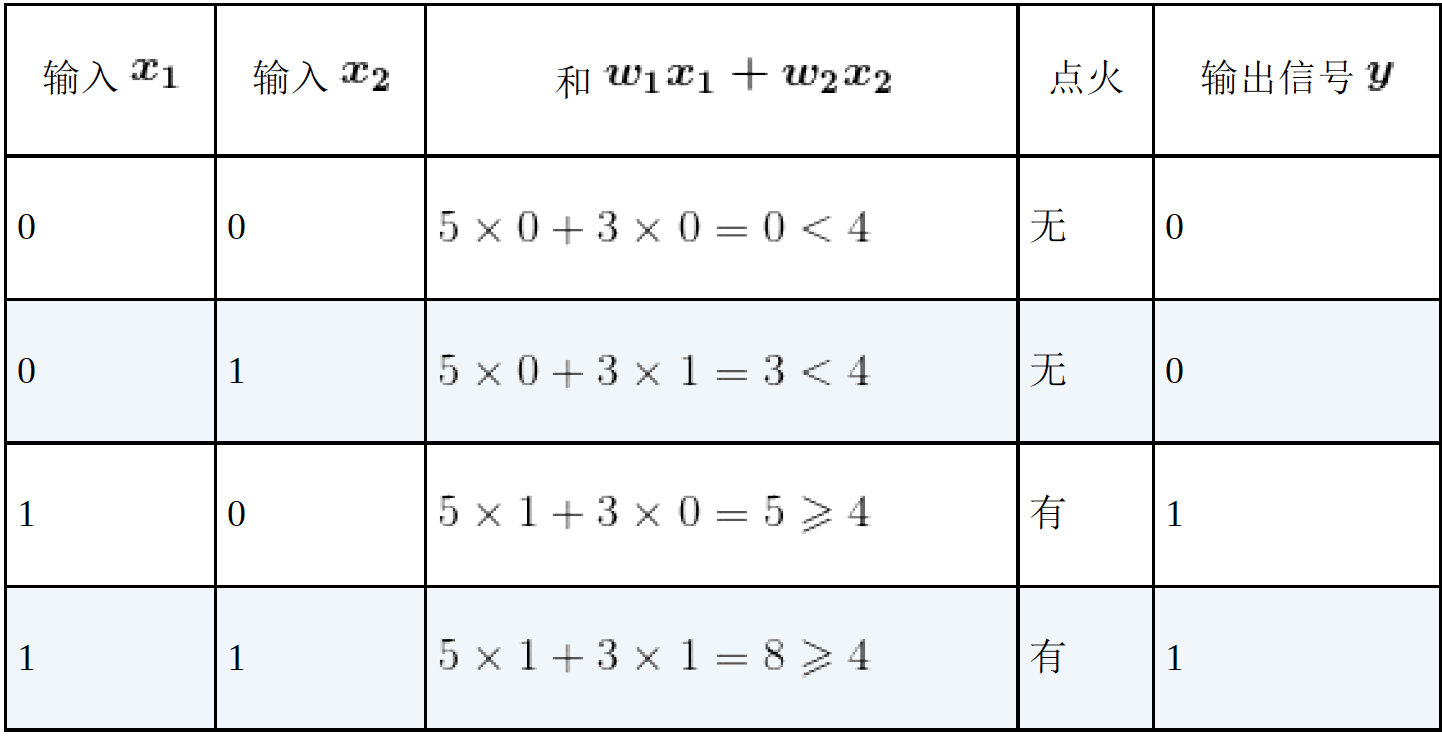## 2.5 点火条件图形表示（阶跃函数）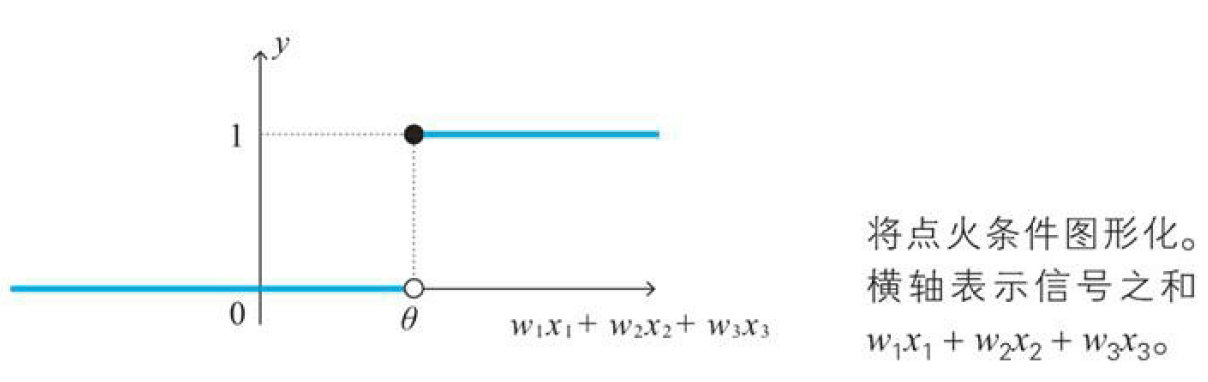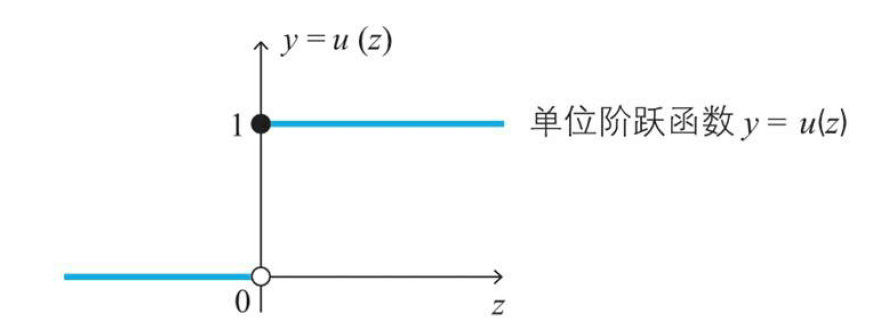## 2.6 激活函数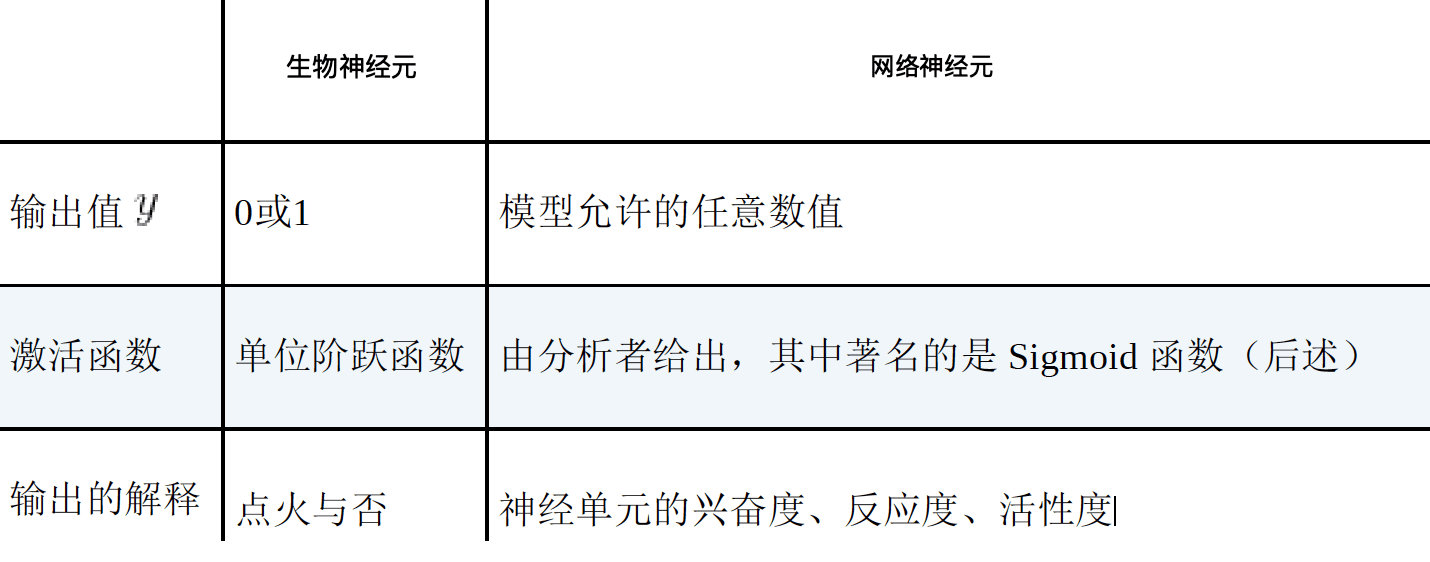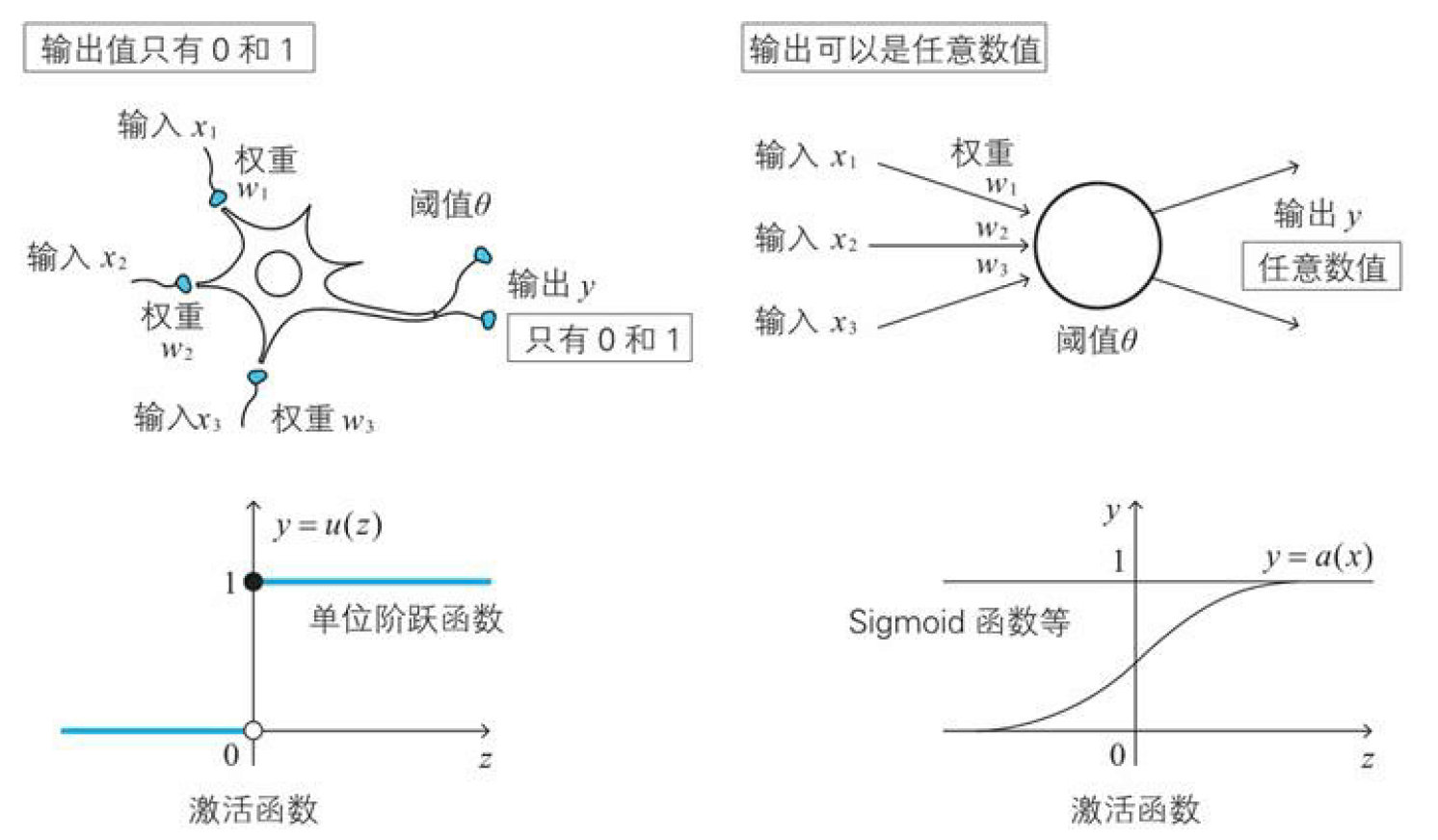### sigmoid函数

$$\sigma (z)=\frac { 1 }{ 1+{ e }^{ -z }} (z\approx2.718281… )$$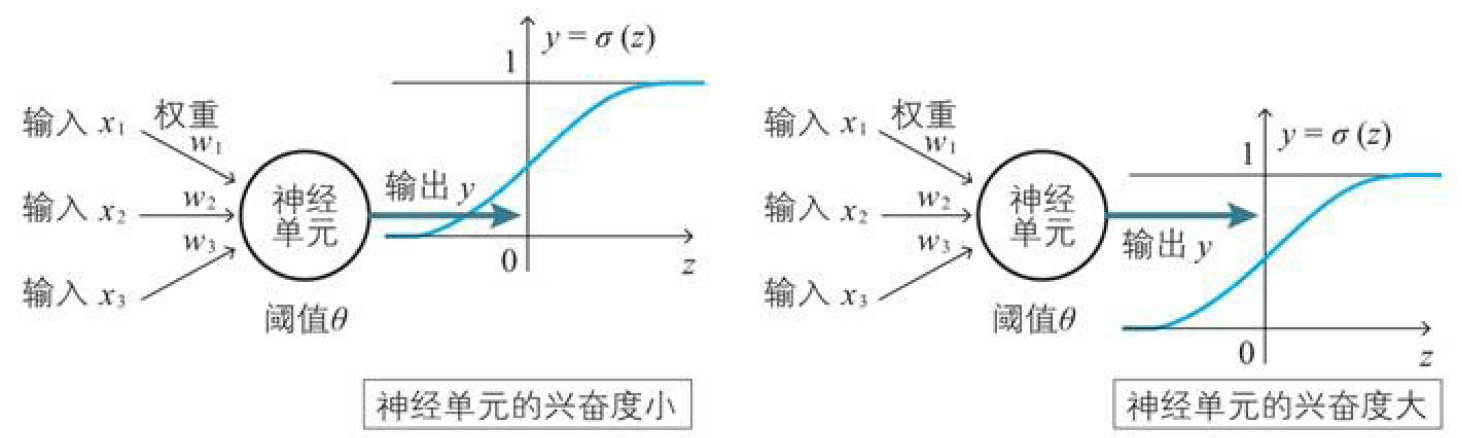## 2.7 偏置

$$z=w_1x_1+w_2x_2+w_3x_3+b$$ $$y=a(z)=a(w_1x_1+w_2x_2+w_3x_3+b )$$